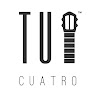TuCuatro

# Seventh Figures: A7#### TuCuatro

The A7 figure, sometimes referred to as the triangle due to its shape when marked on the cuatro, is a simple chord to play in its first position when marking the A7 chord. However, it can be more challenging to apply this figure to the rest of the instrument. This is mainly because the pinky finger must be placed in an unnatural position in order to properly play the chord. Let’s take a closer look at some chords that use the A7 figure:

### A 7 Figure Applied

We can use the same theory for the 7th chords as we do for major chords, such as the D Major chord. To play a different chord based on a different note, simply slide the bar that you use with your index finger down the desired number of frets or half steps until you reach the desired note. For example, let’s say we want to play the B7 chord. We know that the distance between A and B is two-half steps. If we imagine an invisible bar starting from the nut at the top of the cuatro and apply the A7 chord, we can slide this bar down one fret to get the A7# chord, and then down one more half step to reach the B7 chord in its second position.

### A7 to B7 Illustration

0.2.1.2 0.1.0.0

Take a look at how this is applied to the Cuatro below:

### B7 2nd Position

2.4.3.4

If we were to apply the same concept to find other chords, then essentially, you will be able to play any 7th chord with the Cuatro providing that there is enough space to find the note, given that most Cuatros have 15 – 17 frets. Give this a try and find other chords corresponding to other 7th notes using the figure of A7 or triangle. Here is the chord of D7 in its 3rd position to get you started.

### D7 3rd Position

5.7.6.7

Do you want to keep track of your progress? Register an account now to mark lessons as complete.

## Course Content

Lesson Content
0% Complete 0/3 Steps
Lesson Content
0% Complete 0/3 Steps
Lesson Content
0% Complete 0/4 Steps